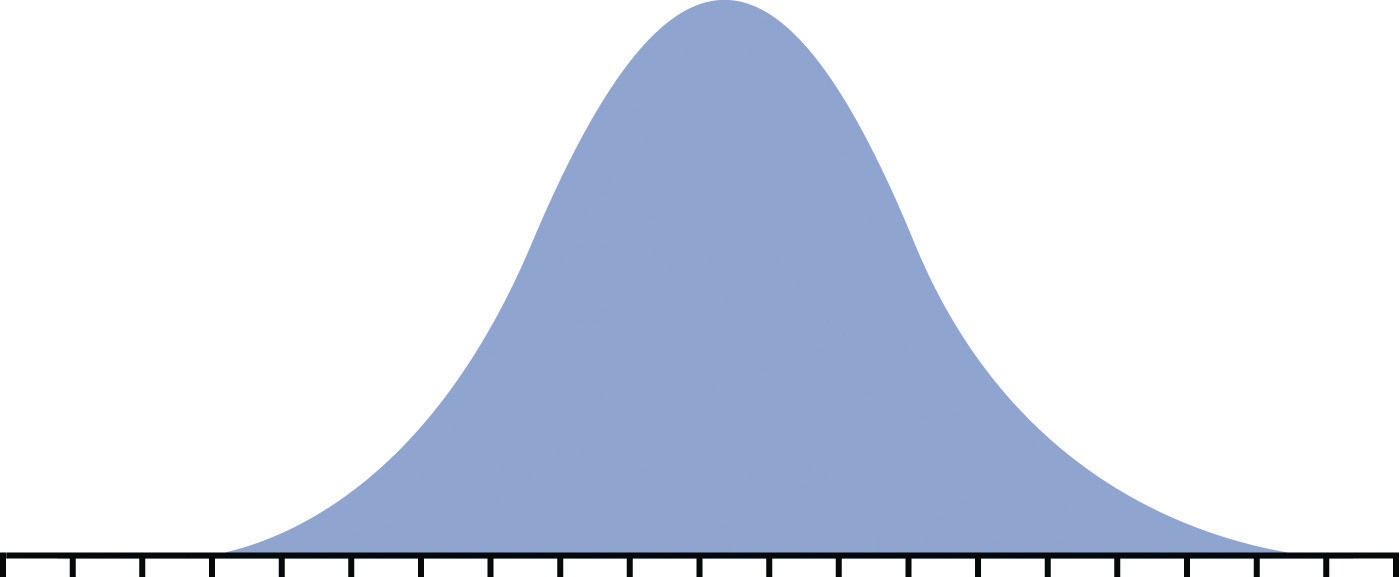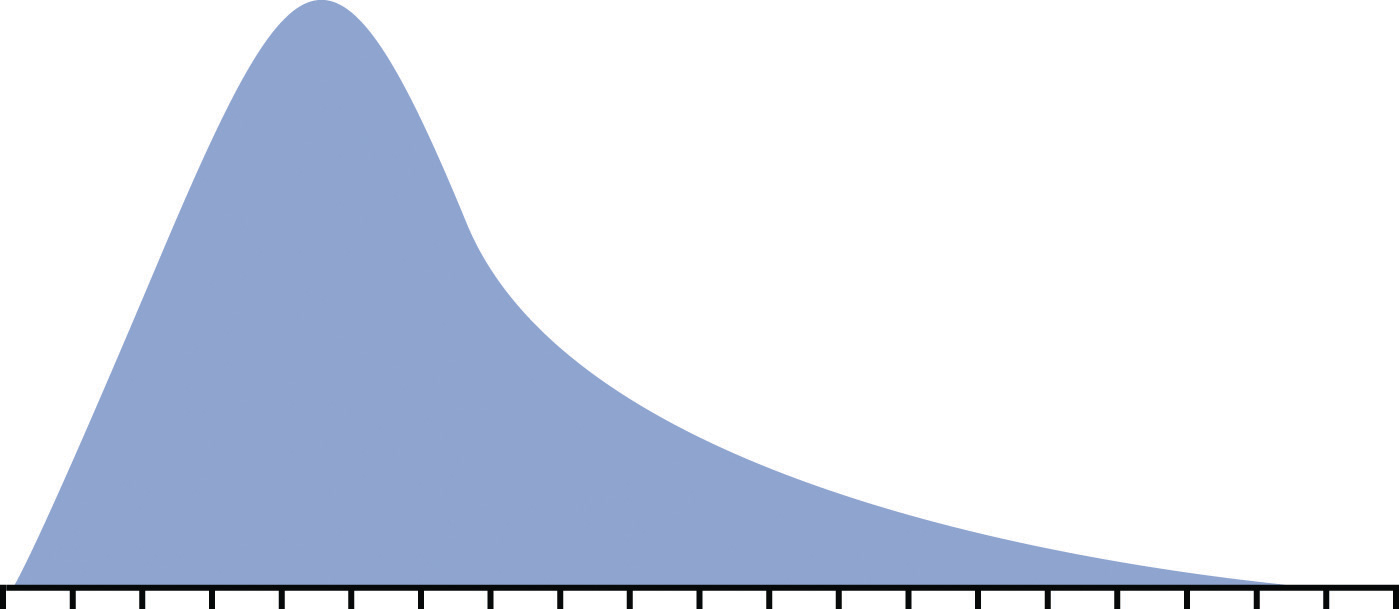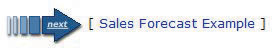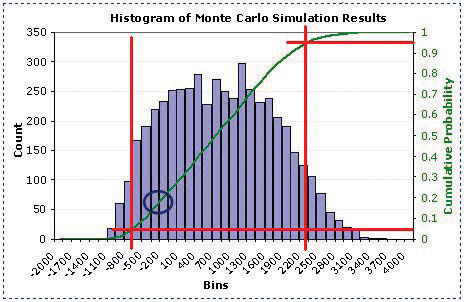## 11.6 Web Exercise

### Learning Objective

1. Describe the benefits of estimating risk using a Monte Carlo simulation.

## Monte Carlo Risk Simulations

Planning for risks is a form of betting on the future. An accomplished gambler knows the odds of drawing a certain combination of cards in a poker hand or of a ball landing on a number at a roulette wheel. If a project has several risk factors, they are not likely to all occur on the same project, but it is important to know the odds of that happening and to compare them to the potential profit of the project. If several risks do materialize on the same project, it might cause the company to lose money on the project, and senior management must decide if the benefit is worth the risk.

Computers can generate random numbers that can be used to simulate the likelihood of combinations of risk factors occurring and the impact on the project’s profitability. These simulations calculate odds like those a gambler would use before placing a bet, and the process is named after a famous gambling center in Europe.

To use a Monte Carlo simulation, you have to decide how the frequency of occurrences is distributed. Three types of distributions are most common: normal, skewed, and equal. If they are governed by the central limits principle, the occurrences will have a normal distribution.

Figure 11.7 Normal DistributionIf the likely frequency of occurrences of a risk factor is more likely to be distributed to either side of the middle of the range, it is a skewed distribution.

Figure 11.8 Skewed DistributionIf the likelihood of occurrence is evenly distributed across the range where each possibility has the same odds of occurring, it is an equal distribution.

Figure 11.9 Equal DistributionA computer can choose numbers for each risk factor that represent a possible outcome for that risk on the project according to its distribution. Those numbers are fed into a spreadsheet that determines the effect on the project and its costs. This process is repeated thousands of times, and the result of each iteration—repeated process—is stored in a table of possible outcomes. This table is summarized in a histogram that shows how many of the iterations produced profit (or loss) in each range (bin).

The outcome of a Monte Carlo simulation gives managers an idea of how much the project could make or lose and the odds of that happening. Monte Carlo simulations are often used to predict the likelihood of a new product making a profit or loss. The same methods can be applied to predicting the profit or loss on a project.

Complete the exercise by following these instructions:

1. Open a blank document in a word processing program and then save the document as Ch11MonteCarloStudentName.doc. Leave the document open.
2. Start a web browser and then to go to A Practical Guide to Monte Carlo Simulations at http://www.vertex42.com/ExcelArticles/mc/MonteCarloSimulation.html.
3. Read the first screen to review the concepts.
4. Near the bottom of the first screen, click the arrow labeled Sales Forecast Example, as shown in Figure 11.10 "Next Page Button".

Figure 11.10 Next Page Button5. Scroll down past the advertisements and begin reading at Step 1. Capture a screen that shows Step 1 and paste it into Ch11MonteCarloStudentName.doc.
6. Read the explanation of how to create a model.
7. Use the Next button at the bottom of the screen to go to step 2, Generating Random Inputs.
8. Read steps 2, 3, and 4 on this screen.
9. Continue reading and advancing screens until you get to the histogram as shown in Figure 11.11 "Estimated Loss or Profit".

Figure 11.11 Estimated Loss or Profit10. The green line is the cumulative probability. The red lines are intended to help you find the 5 percent and 95 percent probability points on the green line.
11. Capture this screen and paste it into Ch11MonteCarloStudentName.doc.
12. Refer to Figure 11.11 "Estimated Loss or Profit". Notice a spot on the green line is circled. According to the horizontal scale, this is the spot on the cumulative percentage line that marks the difference between negative and positive income for the project. In the word processing document, below the last screen capture, describe how you would use this chart to predict the percentage chance that this project will lose money. Leave the document open.

## Learn about Using Dedicated Monte Carlo Simulation Software

Complete the exercise by following these instructions:

2. Read each of the first seven screens. Capture screens where indicated in the following list and paste them into Ch11MonteCarloStudentName.doc:

• Introduction (Capture the section titled The Flawed Average Model.)
• Introducing Uncertainty
• Introducing Uncertainty (cont.)
• Uncertain Functions and Statistics
• Using Interactive Simulation (Capture the table near the bottom.)
• Viewing the Full Range of Profit Outcomes
• Focusing on Profitable Outcomes (Capture the simulation results histogram at the bottom of the screen.)
3. The authors make the case that a simple average of the risks produces an estimate that is too high. If they run a thousand combinations of risk outcomes, they predict a lower profit and a certain likelihood of losing money. In the word processing document, below the last screen capture, review the screens and answer the following questions:

• What does a simple average model predict for a net profit?
• What does the simulation predict is the “True Average” profit?
• If most of the risk factors occur, how much money could the project lose?

## Analysis

1. At the bottom of Ch11MonteCarloStudentName.doc, write between one hundred and two hundred words to describe the benefits of estimating risk using a Monte Carlo simulation versus a simple average of the risks. Use specific references to the assigned reading in the text and in the web pages in the previous two parts of this exercise.
2. Review your work and use the following rubric to determine its adequacy: